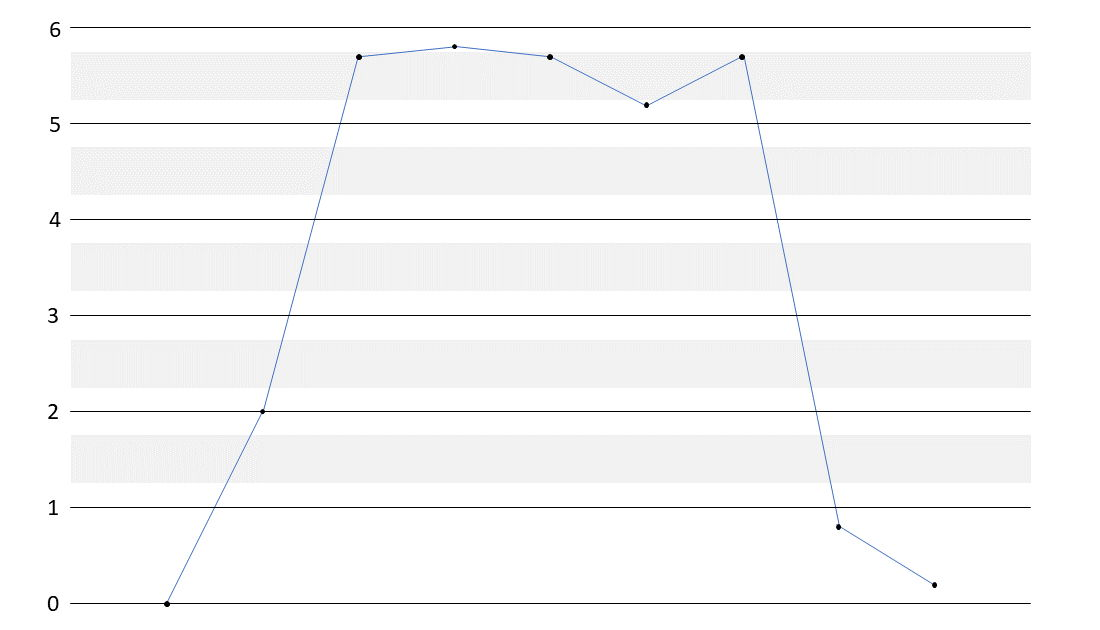시간 제한 메모리 제한 제출 정답 맞은 사람 정답 비율
1 초 (추가 시간 없음) 1024 MB 4 2 2 50.000%

## 문제

A digital speedometer shows a vehicle's speed as integer miles per hour.  There are occasions when the sensed speed varies between two integer values, such as during cruise control.  Using a single threshold to round between adjacent integers often makes the display toggle rapidly between the two integers, which is distracting to the driver.

Your team must implement a smoothing technique for the display using separate rising and falling thresholds ($t_r$ and $t_f$, $t_f < t_r$, respectively). See Figure 1 for a graphical depiction of the Sample Input for use with the following rules.Figure 1: Sensor reading from the Sample Input, with $t_f=0.25$ and $t_r=0.75$.

Each sensed speed, $s$, falls between two adjacent integers $i$ and $j$, $i \le s < j$, where $j = i + 1$.  When displaying the sensed speed $s$ as an integer:

• When $s$ falls between $i$ and $i+t_f$, $s$ is displayed as $i$.
• When $s$ falls between $i+t_r$ and $j$, $s$ is displayed as $j$.
• When $s$ falls between $i+t_f$ and $i+t_r$, $s$ is displayed as $i$ if the most recent preceding value for $s$ outside of range $[i+t_f, i+t_r]$ is less than $i+t_r$, and $s$ is displayed as $j$ if the most recent preceding value for $s$ outside of range $[i+t_f, i+t_r]$ is greater than $i+t_r$.
• Any sensed speed, $0 < s < 1$, must display as $1$ because any non-zero speed, no matter how small, must display as non-zero to indicate that the vehicle is in motion.

## 입력

The first line of input contains $t_f$, the falling threshold. The second line of input contains $t_r$, the rising threshold. The speed sensor reports $s$ in increments of $0.1$ mph.  The thresholds are always set halfway between speed increments. All remaining lines until end-of-file are successive decimal speeds, $s$, in miles per hour, one speed per line. The third line of input, which is the first measured speed, will always be $0$. There are at most $1000$ observed speeds $s$ in input. $$0 < t_f,t_r < 1; \ \ \ \ t_f < t_r; \ \ \ \ 0 \le s \le 120$$

## 출력

Output is the list of speeds, one speed per line, smoothed to integer values appropriate to $t_f$ and $t_r$.

## 예제 입력 1

0.25
0.75
0
2.0
5.7
5.8
5.7
5.2
5.7
0.8
0.2


## 예제 출력 1

0
2
5
6
6
5
5
1
1


## 힌트

Input Output Explanation
0.25   Value of $t_f$.
0.75   Value of $t_r$.
0 0 Initial input.
2.0 2 Input greater than $0$, below threshold of $2.25$.
5.7 5 Input greater than $2.0$, in threshold range.
5.8 6 Input greater than $2.0$, exceeds upper threshold of $5.75$.
5.7 6 Input less than $5.8$, in threshold range.
5.2 5 Input less than $5.8$, below threshold of $5.25$.
5.7 5 Input greater than $5.2$, in threshold range.
0.8 1 Input greater than $0$ and less than $1$.
0.2 1 Input greater than $0$ and less than $1$.

## 출처• 문제를 만든 사람: Ed Skochinski